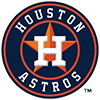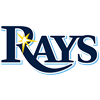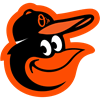2022 AL MVP Odds
-455
0.22 to 1Aaron Judge
70.1% implied probability

+350
3.5 to 1Shohei Ohtani
19.0% implied probability

+5000
50 to 1Yordan Alvarez
1.7% implied probability

+6600
66 to 1Jose Ramirez
1.3% implied probability

+10000
100 to 1Vladimir Guerrero
0.8% implied probability

+10000
100 to 1Rafael Devers
0.8% implied probability

+20000
200 to 1Tim Anderson
0.4% implied probability

+20000
200 to 1Jose Altuve
0.4% implied probability

+20000
200 to 1Xander Bogaerts
0.4% implied probability

+20000
200 to 1Kyle Tucker
0.4% implied probability

+25000
250 to 1Shane McClanahan
0.3% implied probability

+25000
250 to 1Julio Rodriguez
0.3% implied probability

+25000
250 to 1Anthony Rizzo
0.3% implied probability

+25000
250 to 1Justin Verlander
0.3% implied probability

No changes have been recorded yet.
+25000
250 to 1Byron Buxton
0.3% implied probability

+50000
500 to 1Teoscar Hernandez
0.2% implied probability

+50000
500 to 1Mike Trout
0.2% implied probability

+50000
500 to 1Ty France
0.2% implied probability

+50000
500 to 1J.D. Martinez
0.2% implied probability

+50000
500 to 1Cedric Mullins
0.2% implied probability

+50000
500 to 1George Springer
0.2% implied probability

+50000
500 to 1Luis Robert
0.2% implied probability

+50000
500 to 1Bo Bichette
0.2% implied probability

+50000
500 to 1Marcus Semien
0.2% implied probability

+50000
500 to 1Trey Mancini
0.2% implied probability

+50000
500 to 1Carlos Correa
0.2% implied probability

+50000
500 to 1Corey Seager
0.2% implied probability

+50000
500 to 1Alex Bregman
0.2% implied probability

+50000
500 to 1Eugenio Suarez
0.2% implied probability

+50000
500 to 1Gleyber Torres
0.2% implied probability

+50000
500 to 1Gerrit Cole
0.2% implied probability

+50000
500 to 1Giancarlo Stanton
0.2% implied probability